# 1.8 Fractions-Incomplete Measure

## 1.8 Fractions-Incomplete Measure

When counting discrete units, every natural number, such as 1, 2, 3, 4, etc., is known by its ratio to 1. For example, 2 is twice as large as 1, 3 is three times 1, 4 is four times greater than 1, and so on. By way of ratio, the relationship between any two quantities can be expressed in terms of how much larger the first is to the second quantity, and how much smaller the second is to the first quantity. In this section, fractions are used to measure continuous data and to express amounts that are less than a whole discrete unit. Consider the following number line: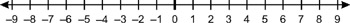Figure 1.3

The line has been split into a number of divisions, and we will now consider 1 and any other number in a continuous way as opposed to a discrete way. Each division along the line is the same length: 1, 2, 3, and so on. There is a point, however, that is halfway to 1-this point is less than 1 and is therefore some lower value between 0 and 1. Likewise, I might not choose a point halfway but a quarter of the way along, or perhaps a third. In all cases, it is possible to subdivide the space between any two numbers and break it into smaller pieces that are not themselves a complete unit. To express this measurement we use fractions.

Fractions are commonly called vulgar fractions or common fractions. Each fraction has two terms that correspond to the terms of a ratio and are separated by a horizontal line (called a vinculum). There is a number on the top (numerator) and a number below (denominator). Fractions come in three forms: proper fractions, mixed numbers, and improper fractions. The following sections discuss these forms.

• Proper fractions-Proper fractions express a number less than 1. In other words, the numerator is less than the denominator. Consider the number line in Figure 1.3. The point halfway between 0 and 1 (half of 1) is called ½. This is a proper fraction, and 1 is the numerator and 2 is the denominator.

Just as we know every number by its ratio to 1-for example, 2 is twice 1, 3 is three times 1, etc.-we must also understand proper fractions in terms of their relation to 1. The fraction ½ is half of 1, and 1 is two times ½. This fraction is therefore called ½ ("one half") because of the ratio of 1 to 2. 1 is one-half of 2, and 2 is two times 1.

From this it can be established that fractions are a ratio, and just like every number they have a ratio to 1. The two terms of the fraction, the numerator and the denominator, are in a ratio, and the fraction overall also has a ratio to 1. This can be stated more formally by saying that ½ has the same ratio to 1 as the numerator does to the denominator. These two ratios are therefore proportional and are expressed as:

This rule applies to all fractions. For example, ¼: 1 = 1:4, ¾: 1 = 3:4, and so on.

• Mixed numbers-Mixed numbers are a combination of a whole number and a proper fraction. For example, 2½ ("two and a half") means 2 plus ½.

• Improper fractions-Improper fractions are greater than or equal to 1, which means the numerator is greater than or equal to the denominator. They are sometimes called top-heavy fractions. Examples are: 3/3, 9/7, or 45/3. Because it can be difficult to visualize improper fractions, they can be reduced to mixed numbers.

### 1.8.1 Unit and Reciprocal Fractions

Unit fractions are called the reciprocal of a number because they are the opposite. For example, the reciprocal of 2 is ½, the reciprocal of 4 is ¼, and so on. The reciprocal of any number then means making that number the denominator of a fraction and making the numerator 1. In short, a unit fraction is a fraction that has a numerator of 1.

 Note Division by a number, say 2, is the same as multiplication by its reciprocal. So 10 ÷ 2 is the same as 10 × ½.

It is also important to remember that a fraction's ratio to 1 is the same as the numerator's ratio to the denominator. In terms of a part and parts of numbers as discussed earlier, it can be useful to see unit fractions as follows in terms of their relationship to 1:

• ½ (one half) is half of 1.

• (one third) is the third part of 1.

• ¼ (one fourth, one quarter) is the fourth part of 1.

There are also proper fractions such as and ¾ where the numerator is greater than 1. These are named according to the ratio between the numerator and denominator, just like any other fraction. ¼ is one fourth part, or one quarter. ¾ is therefore three of them-¼ + ¼ + ¼-and this is three fourth parts, or three quarters. More specifically, it is three fourth parts of 1.

### 1.8.2 Fractions and Whole Numbers

If the reciprocal of 2 is ½, then the reciprocal of ½ must be 2. For this reason, any number can be expressed as a fraction as itself over a denominator of 1. For example, 2/1 is the reciprocal of ½ (the numerator and denominator are swapped). This makes sense since 1 divides into 2 twice. This is because of the ratio of the numerator to the denominator: 1 is half of 2 and 2 is two times 1. Here are some reciprocals:

Alternatively, a whole number can be written as an improper fraction of any denominator by multiplying itself by the denominator and writing the product as the numerator. For example, 2 can be expressed as a fraction over a denominator of 8 as follows:Note A whole number, such as −1, 0, 1, 2, and so on, is called an integer.

### 1.8.3 Complements

Proper fractions represent a number less than 1, and this means their numerator is less than their denominator. Fractions are therefore a part of 1, as we have seen. ½ is one half of 1, ¾ is three fourths of 1, and so any proper fraction can be considered some part of 1. If, given a proper fraction, you are asked to find its complement, then it means you need to find a second fraction which, when added to the first, will equal 1. In other words, if two fractions are added and their sum is 1, they are said to be complements because they are two parts of a whole.½ + ? = 1 (One half plus what number equals 1?)

The complement of ½ is ½ because two halves make one whole item. 1 is two times ½; therefore two halves equal 1.Note Another way of asking for the complement of a fraction is to ask a subtraction question: 1 − ½ = ½.

### 1.8.4 Equivalents

It was stated earlier that when each term of a ratio is multiplied by the same number the result is an equivalent ratio; that is to say, the same ratio. 3: 15 multiplied by 3 is 9: 45; both of these are the same ratio and both these ratios can be reduced to lowest terms in the ratio 1: 5.

This idea of equivalents also applies to fractions since fractions represent the ratio between the numerator and the denominator. Consider the pie chart below. It is divided into eight equal segments, and therefore any fraction of this pie can be represented as a numerator over 8 (the denominator). Since there are eight equal divisions, if I eat one slice then I have eaten , and there are (seven eighths) remaining. Notice also how these two fractions ( and ) are comxsplements; if they were added together they would equal 1.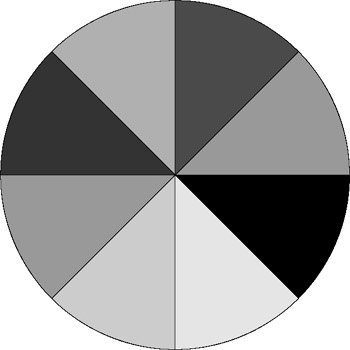Figure 1.4

In the context of the pie with eight equal pieces, the following two fractions are equivalent; that is, they represent the same value. We know this because the numerators and denominators of both fractions are in the same ratio: 4/8 and ½.

In each of these fractions, the denominator is twice as much as the numerator, and the numerator is half the denominator. Thus, 4 is to 8 as 1 is to 2, and because of this each fraction is in the same ratio when related to 1. Both fractions, then, are half of 1.

The fraction 4/8 is not expressed in its lowest terms, while ½ is the same fraction but expressed in lowest terms. The method of reducing a fraction to its lowest terms is the same as that for reducing a ratio to its lowest terms; this is the theory of the common divisor, as demonstrated previously. This process involves finding a number that both terms can be divided by because, just as multiplying both terms by the same number results in an equivalent fraction, so does dividing both terms by the same number. Like a ratio, a fraction is said to be in its lowest terms when the numerator and denominator have no common divisors except 1. The following fraction has been reduced several times.

### 1.8.5 Comparing Fractions

It is useful to compare fractions and determine which is smaller and which is larger, and there are a number of ways to do so.

• Comparing fractions with the same numerator

For example, which is larger out of ½ and ¼? In comparing fractions with equal numerators, the fraction with the larger denominator (in this case ¼) is always the smallest, while the fraction with the smaller denominator is always the larger of the two numbers.

• Comparing fractions with the same denominator

Which is greater: ¼ or ¾? If the numerators differ but the denominators are the same, then the larger fraction is the one with the greater numerator. In this case, ¾ is larger than ¼ because 3 is greater than 1.

• Comparing different fractions

Which is greater: ½ or ¾? If the denominators of two fractions differ, then one or both of the fractions must be made into equivalent fractions so each fraction has the same denominator. To make an equivalent fraction, we must multiply both the numerator and denominator by the same whole number. So, we must multiply one or both of our fractions by a whole number, and the resulting fraction must have a denominator that is equal to the other fraction's denominator. In this example, which number can ½ be multiplied by that will give us a resulting fraction with 4 as a denominator? In order to find this number, we must find the LCM, which stands for lowest common multiple.

 Note The lowest common multiple of two numbers is the first multiple of both numbers that coincide. For example, here are some multiples of 3 and 5: 3, 6, 9, 12, 15 5, 10, 15 Here, the LCM of 3 and 5 is 15 because it is the first multiple that both 3 and 5 have in common. In short, to find the LCM between any two numbers, cycle through the multiples of the larger number (including the larger number itself) and see where its multiples coincide with multiples of the smaller number. When calculating the LCM, if the given numbers are in their lowest terms (in other words, they can be divided by no common number except 1), then their LCM is their product. 3 and 5 are in their lowest terms, and their LCM is also the product of 3 and 5 (3 × 5 = 15).

For example, let's compare ½ and ¾. First, we need to find a common denominator.

The denominators are 2 and 4.4 is a multiple of 2. Therefore, 4 is the LCM.

Make an equivalent fraction of ½ such that it shares the denominator of 4. Both the numerator and denominator must be multiplied by the same number to make an equivalent fraction. This number must be 2, because 2 × 2 = 4. So: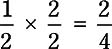Now we can compare the fractions 2/4 and ¾.¾ is the larger number.

### 1.8.6 Adding and Subtracting Fractions

Just as any whole numbers can be added and subtracted, so can fractions. In order to add or subtract a fraction with the same denominator, add or subtract only the numerators, leaving the denominators the same. Any two fractions with different denominators must be made into equivalent fractions with equal denominators using the LCM, as demonstrated in the previous section.

For example: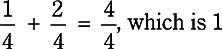Note Adding mixed numbers follows the same rule. Add the two whole numbers and then add the fractions.

### 1.8.7 Multiplying and Dividing Fractions

Fractions can also be multiplied and divided. There are several kinds of multiplication and division operations on fractions, which are described here.

• Multiplying fractions by fractions-Multiplying two fractions means multiplying the numerator of one fraction by the numerator of the other, and multiplying the denominator of one by the denominator of the other. In short, multiply corresponding terms.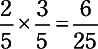• Multiplying fractions by whole numbers-Multiplication is repeated addition. We know that 3 × 3 = 3 + 3 + 3 = 9. Therefore, to multiply a fraction by a whole number, the following must occur:

We know this is true because multiplication is repeated addition, and addition of fractions does not change the denominators, only the numerators. Alternatively, a whole number can be represented as a fraction as being a numerator over 1. In this case, the rule of fraction by fraction multiplication holds, but the result is still the same, as follows: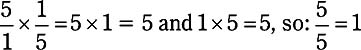Note You should notice that in terms of ratio 1 is one fifth part of 5, because 5 is five times 1. The result of multiplying 5 by ⅕ gives the answer 1. Multiplication of a whole number by a fraction therefore can be seen as leading to a part of the whole number. ½ × 2 is 1 because ½ of 2 is 1, and therefore 2 is twice as large as 1. Likewise, ⅓ of 3 is 1, and ¼ of 4 is 1. ½ of 4 is 2, and so on.
• Multiplying fractions by mixed numbers-Fractions can be multiplied by mixed numbers when the mixed numbers are converted into improper fractions. Improper fractions are fractions greater than one, and we have seen how they correspond to mixed numbers.

3 ¾ as an improper fraction is 15/4. This is because one whole is composed of four divisions. There are four ¼ (quarters) in a whole unit. There are already three whole units, so a total of 4 × 3 quarters is 12 quarters. This is added to the numerator of the fraction to make 15. So:

• Division by the same denominators-It can sometimes be awkward to imagine exactly what it means to divide a fraction by another fraction: ½ ÷ ½ = 1 means that ½ fits into ½ exactly once. One method of dividing fractions is to make sure both denominators of the dividend and divisor fractions are the same. If the denominators are different, then the LCM must be found to change the fractions into equivalents with the same denominator. Once two fractions have the same denominator, only the numerators are divided, as in addition. Division can be written as a fraction. So:

• Division by inverted multiplication-It was mentioned in previous sections that division by a number can be seen as multiplication by its reciprocal. For example, 2 ÷ 2 is the same as 2 × ½. 2 can be expressed as the fraction 2/1, and its reciprocal is ½. This can also apply to fraction multiplication.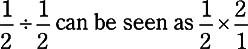Following the standard rules of fraction multiplication as mentioned earlier, corresponding terms are multiplied. So: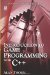Introduction to Game Programming with C++ (Wordware Game Developers Library)
ISBN: 1598220322
EAN: 2147483647
Year: 2007
Pages: 225
Authors: Alan Thorn

Similar book on Amazon

flylib.com © 2008-2017.
If you may any questions please contact us: flylib@qtcs.net